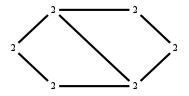# The Markov Bases Database

## G128_bin

The binary graphical model/graph model of G128.

It is a hierarchical model of 6 variables. The dimension of the model is 13.The cardinality of the statespace is 64.

### Properties of the Markov basis

 Markov degree 4 2390

 degree # of gen. 2 4 384 2006

The model has the following properties:

• All variables are binary.
• It is a graph model and a graphical model.
• The semigroup is normal.

The following properties of the model are unknown:

• The minimal Markov basis may be unique.### FILES

 Markov basis: sufficient statistics matrix: G128_bin.mar (450.47 kb) G128_bin.mat (3.53 kb) G128_bin.mod (59 b) G128_bin.tar.gz (20.57 kb)

Wrong or missing information? Write us an email.

page last update on November 15 2020. ->Contact